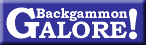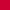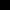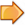Cube Theory# On Optimal Doubling in Backgammon Norman Zadeh and Gary Kobliska, 1977

 Originally published in Management Science, vol. 23, no. 8, April 1977.

Abstract. The concept of an effective doubling number for noncontinuous games is introduced. Computer simulation is employed to determine an extremely accurate strategy for accepting, doubling, and redoubling in running games.

## Introduction

Backgammon is a two-player perfect information game in which a player’s move is governed by a throw of the dice. A player throws the dice and then moves, after which his opponent throws and moves, etc. If a player rolls, say, 5 and 3, then he may move one man 5 spaces and another man 3 spaces, or one man 8 spaces. Towards the end of the game, a race usually develops with the winner being the player who takes his men off first. Race situations may be accurately approximated by the following contest involving two players, A and B.

A rolls first and starts out xA spaces from home, while B begins as distance xB from home. (xA is called player A’s count). Each player’s count is reduced by the total of his roll. Thus if A’s count is 45, and he rolls 5 and 3, his count becomes 37. Player A wins if and only if his count becomes ≤ 0 before B’s count becomes ≤ 0.

Since gammons and backgammons (these are bonuses) rarely occur in running games, they will be disregarded.

Players are allowed to double the stakes if they follow betting rules which will be explained in the next section. We give the optimal doubling and redoubling strategies for race-type positions. The strategies we present differ from those given by Keeler and Spencer (Keeler et al. 1975) because they assumed that the total of a player’s dice could be 5 with probability 11/24, 10 with probability 11/24, and 20 with probability 2/24. In reality, for race purposes, the dice can show any one of 13 numbers.

## Continuous Games

Let p(t) denote the probability that player A will win at time t (given both players play optimally.) If p(t) is a continuous function of time, we call the game continuous. In backgammon, p(t) is not continuous since it is defined only on nonnegative integers and may jump considerably from one move to the next. We begin by assuming that p(t) is continuous and then make modifications to adjust for its actual discontinuity. The following lemma is from Keeler and Spencer (Keeler et al. 1975). Observe that A wins if p(t) becomes 1 before it becomes 0.

Lemma 1.  In a continuous game, let the probability of A winning be x, and let a, b > 0 be such that 0 ≤ x − a < x < x + b ≤ 1. Let E be the event that p(t) becomes x + b before it becomes x − a. The the probability of E is a/(a + b).

Proof.  We have, by conditioning on whether p(t) first reaches x + b or xa, x = p(E) · (x + b) + (1 − p(E)) · (xa). This yields

 p(E) = x − (x − a)(x + b) − (x − a) = aa + b .

Example 1.  Suppose that x = 0.75. Then the probability that A wins (p = 1 before p = 0) is 0.75/1 = 0.75.

Example 2.  Suppose that x = 0.6. Then the probability that x = 0.8 before x = 0 is 0.6/(0.6 + 0.2) = 3/4.

Lemma 1 may be extended to any linear function of p(t), such as A’s expectation. In other words, the probability that A’s expectation rises by b before it falls by a is also a/(a + b).

## Doubling

In backgammon, the stakes of the game are determined by the doubling cube, which is initially put in the middle of the board with the “1” face up, meaning that the game is initially worth 1 point. During the course of a 1-point game, either player may double the stakes before rolling his dice. If A doubles to 2, then B may either concede 1 point to A and start a new game or agree to play the existing game for 2 points. If B elects to play on then only he may redouble. (All doubles after the first are called redoubles.) Suppose that B decides to play and eventually redoubles to 4. Then A may either give B 2 points and start a new game, or play for 4 with the sole right to redouble, etc. There is no limit to the number of redoubles.

It is shown in Keeler and Spencer (Keeler et al. 1975) that in a continuous game, A should double or redouble when p = 0.8. When A doubles it does not matter whether B accepts or declines, his expectation in both cases is −(the current value of the game). To see this, let K denote the value of the game before A doubles. If B accepts, he will own the cube at 2K. He will win when p = 0.2 and lose when p = 1. Using Lemma 1, his probability of winning is 0.2/(0.6 + 0.2) = 1/4. Therefore his expectation is 1/4·(2K) − 3/4·(2K) = −K, which is what he would lose if he did not accept the double.

## Effective Doubling Point in Noncontinuous Games

Because backgammon is not a continuous game, one cannot wait for exactly an 80% chance of winning before doubling. Some doubles will be made at 70%, 74%; others will be made at 84%, 89%, etc. Note that a player’s expectation after doubling to 2K at 70% is less than K.

In a noncontinuous game, the cube loses some of its value because one might have to reach an 85% position part of the time to win by doubling instead of an 80% position. (Having to reach 70% to double may be shown to be roughly equivalent to having to reach some point > 0.80 to double.) We may measure this loss of value by approximating our discontinuous game by a continuous one with doubling points DA for A and DB for B. Both are functions of the state of the game, i.e., the position of the checkers, whose roll it is, etc. DA lies between 0.80 and 1. DB lies between 0.20 and 0. A wins when p(t) = DA, B wins when p(t) = DB.

The loss of the cube value to A is measured by the magnitude of DA − 0.80. When DA = 0.80, as is the case in a continuous game, the cube has a maximum value since A must travel the minimum distance to win. As DA moves upward, the cube loses value. When DA = 1.0 the cube has no value to A.

In general, as the race nears the end, DA will increase since p(t) will become “less continuous.” In an even race with 40 spaces to go and the cube in the middle, our results indicate that DA ≈ 0.88 and DB ≈ 0.12. With 20 pips to go, DA ≈ 0.90 and DB ≈ 0.10.

Lemma 2.  In a continuous game let DA(t) and DB(t) represent the effective doubling points for A and B at time t. Let p(t) represent A’s chances of winning at t assuming a cube is not used. Let p(t, A) denote A’s chances when holding the cube, and let p(t, B) denote A’s chances when B holds the cube. Let p(t, M) denote A’s chances when the cube is in the middle. Then we have

 p(t, A) = p(t)/DA(t), (1)

 p(t, B) = (p(t) − DB(t))/(1 − DB(t)), (2)

 p(t, M) = (p(t) − DB(t))/(DA(t) − DB(t)). (3)

Proof.  The proof is immediate from Lemma 1. For example, to prove (3), we take x = p(t), a = p(t) − DB(t), b = DA(t) − p(t). Then

a/(a + b) = (p(t) − DB(t))/(DA(t) − DB(t)).

Theorem 1.  In a continuous game with effective doubling points DA(t), DB(t) for A and B respectively, let M(t) denote the greatest value of p(t) for which B can accept A’s double. M(t) is called the minimum take point and varies from 75% to 80%. Let r(t) and d(t) denote the minimum values of p(t) for which A can double and redouble respectively. Then we have

 M(t) = 1 − 1/4·(1 − DB(t)), (4)

 r(t) = 3DA(t)DB(t) + DA(t)4DA(t) − 2(1 − DB(t)) ,
(5)

 d(t) = (DB(t))2 + 3DB(t)(1 − DA(t)) − DA(t)2DB(t) − 4DA(t) + 2 .
(6)

Proof.  We prove (4) first. After A doubles, B’s chances of winning given that he owns the cube are 1 − p(t, B), which by (2) of Lemma 2 equals

 1 − p(t) − DB(t)1 − DB(t) .
(7)

B’s minimum take point occurs when (7) = 25%, i.e., we have

 0.25 = 1 − M(t) − DB(t)1 − DB(t) .

Solving this for M(t) yields (4).

Proof of (5). r(t) is determined by the condition that A’s expectation after redoubling be equal to A’s expectation before redoubling. A’s expectation before redoubling equals

p(t, A)K + (1 − p(t, A)) (−K) = K(2p(t, A) − 1).

By (1) of Lemma 2, this equals

 K(2p(t)/DA(t) − 1). (8)

A’s expectation after doubling is

 2K(2p(t, B) − 1), (9)

which by (2) of Lemma 2 equals

 2K ( 2p(t) − DB(t))1 − DB(t) − 1 ) .
(10)

When p(t) = r(t), we must have (8) = (10), or

 2(r(t))DA(t) − 1 = 2 ( 2(r(t) − DB(t))1 − DB(t) ) − 1.
(11)

Solving (11) for r(t) yields (5).

The proof of (6) is similar to the proof of (5) and involves equating 2p(t, M) − 1 to 2(2p(t, B) − 1) and solving for p(t).

## Optimal Doubling and Redoubling Strategy for Running Games

Recall that a running game may be approximated by a contest between two players A and B with counts xA and xB respectively. We assume that A rolls first. Each player’s distance from home is decreased by the total of his roll. Player A wins if his count becomes ≤ 0 before B’s count becomes ≤ 0. This approximation is extremely accurate provided that neither player has gaps in his home board or men on the one and two points.

Player A wins if, when he takes n rolls to get off, B takes at least n rolls. Let P(x, n) denote the probability that a player with count x gets off in exactly n rolls. Then the probability that A wins is simply

 ∞∑j=1 ( P(xA, j) ∞∑k=j (P(xB, k) ).

This quantity may be calculated exactly using convolutions. Let f(y) denote the probability that a player’s total in one roll is y. In backgammon, the minimum roll is 3 and the maximum roll is 24. Thus we have

 P(x, 1) = 24∑y=x f(y).

P(x, k + 1) may be computed using the recursion

 P(x, k + 1) = 24∑y=3 f(y) · P(x − y, k).

Table 1 was computed using the above method. It gives the probability (in percent) that a player will win when it is his roll and his opponent’s count is at various distances from his own. For example, when A’s count is 70 and he is 10 pips ahead, his probability of winning is 77.9%.

Table 1.  Probability of winning the running game if it is your turn to roll.

 Your count 10 20 30 40 50 60 70 80 90 100 110 Opponent’s  count −20 0.0 0.0 5.1 8.3 11.6 14.2 16.5 18.4 20.0 21.4 22.6 −15 0.0 7.6 13.2 18.0 21.2 23.7 25.6 27.2 28.5 29.6 30.5 −10 0.0 20.3 27.6 31.3 33.6 35.2 36.4 37.3 38.1 38.7 39.3 −5 32.2 44.4 46.5 47.0 47.4 47.7 47.9 48.0 48.1 48.2 48.3 Even 78.2 68.7 64.3 62.2 60.8 59.9 59.1 58.5 58.0 57.6 57.3 +5 90.6 82.9 78.0 74.8 72.4 70.7 69.3 68.2 67.2 66.4 65.7 +10 94.8 91.1 87.2 84.1 81.6 79.6 77.9 76.5 75.3 74.2 73.2 +15 99.3 96.0 93.2 90.6 88.4 86.5 84.8 83.3 81.9 80.8 79.7 +20 99.7 98.3 96.6 94.8 93.0 91.4 89.9 88.5 87.3 86.1 85.0 +25 99.9 99.4 98.4 97.2 96.0 94.8 93.5 92.4 91.3 90.3 89.3 +30 100.0 99.8 99.3 98.6 97.8 96.9 96.0 95.1 94.2 93.4 92.5

Keeler and Spencer (Keeler et al. 1975) computed a similar table by using a method which involved rolling the dice 1,000 times to approximate P(x, n) for all values of x from 1 to 125. It is interesting to note that their results differ from Table 1 by no more than 1%.

## Results of Computer Simulation

To compute the optimal doubling and redoubling strategies and the minimum take points, we began by analyzing racing positions right near the end. One of us (G.K.) had a piece-moving program which was utilized to compute the optimum doubling and redoubling strategies in actual board positions up to about 12 pips. The computer was instructed to follow these strategies (which are best in actual play) even though they might not coincide exactly with the best strategies for the mathematical race model. In other words, the strategies we obtain are slightly more accurate than those which would be obtained by adhering strictly to the model. From 12 to 20 pips we made intelligent guesses as to the best doubling and redoubling strategies. A CDC 6400 was then asked to compare the expectation after redoubling at 20 vs. 20 versus the expectation by holding onto the cube and rolling. Ten thousand games were played out. The results were used to modify some of the guesses slightly using interpolation and new runs were made with the then accurate strategies. This procedure was repeated at 40 pips, 70 pips, and 110 pips. The program was checked by asking it to print out all information for 50 sample games, both with the cube in the middle and with the cube in A’s possession. The results of the run are given in Table 2.

Table 2.  Results of the simulation.

 Count Expectation Player A Player B Not doubling Doubling Redouble 20 20 0.611 0.685 Redouble 20 19 0.470 0.435 Redouble 20 19 0.481 0.472 Double 20 18 0.330 0.313 Redouble 40 44 0.744 0.783 Redouble 40 43 0.693 0.693 Redouble 40 43 0.689 0.681 Double 40 42 0.577 0.580 Redouble 70 78 0.791 0.822 Redouble 70 77 0.766 0.747 Redouble 70 77 0.737 0.721 Double 70 76 0.664 0.652 Redouble 110 122 0.833 0.866 Redouble 110 121 0.818 0.796 Double 110 120 0.757 0.769 Double 110 119 0.710 0.709

All entries in Table 2 assume that A rolls first. The first entry of each pair gives A’s expectation assuming he holds onto the cube for at least one roll. The second entry gives A’s expectation assuming he doubles immediately. The term “double” in the leftmost column means that the cube is initially in the middle. Thus the first set of entries in the table indicate that when A’s count is 20 and B’s count is 20, A’s expectation by holding onto the cube is 0.611 whereas his expectation by doubling before rolling is 0.685.

Table 3.  Optimal doubling strategy in running games.

 Your count 20 40 70 110 Minimum take for opponent +2 +5½ +10 +14½ Redouble −½ +3 +7½ +11½ Double −1½ +2 +6½ +9½

Table 3 is based on Table 2 and gives the optimal strategy for accepting, doubling, and redoubling. The first column indicates that when your count is 20, you should redouble if your opponent’s count is 19½ or more, and double if his count is 18½ or more. Essentially, this means that you should not redouble when his count is 19 unless there is a weakness in his position (a gap or men on the 1 and 2 points, etc.) Your opponent should drop when his count is 23 or higher and take otherwise.

## ReferencesArticles by Norman ZadehArticles by Gary KobliskaArticles on cube theory Return to:  Backgammon Galore